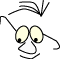JOGL>Torus

# Torus

What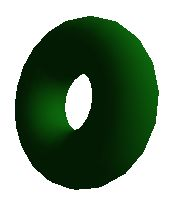Drawing a torus, with and without displaylists

The torus is completely described by the radius of the torus,R, and the radius of the body,r. We want to find parameters that combine the two rotations that are necessary to identify each point on the surface of the torus. One rotation around the main axes and one rotation around the body of the torus.

We will make two different programs for rendering a torus (or two). One is a simple application based on an animator, and the render action in the main class. The other application is based on "a render as needed" philosophy, with an JOGLPanel within a main frame.

Both applications use the same class oneTorus to display a torus and the class stdMaterials to set materials, and both use displaylists to render the torus.

## Torus Geometry

Let w describe the rotation around the main axes, and v the rotation around the torus body. We sketch the torus in two projections: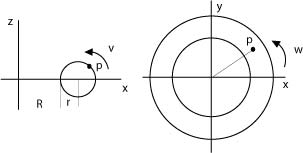When we inspect the illustration we can see that P's coordinates are:

```  z=r.sin(v)
y=(R+r·cos(v))sin(w)
x=(R+r·cos(v))cos(w)
```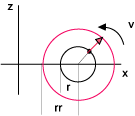That means that we can describe any point on the torus's surface by the three parametric equations above. When we can describe all points, we can also describe all surfaces we want to divide the torus surface into by the same presision.

The subsurfaces on the torus can be generated as polygons where we let the two rotation run a full circle in a double loop. The normal in each point can be found by assuming surrounding torus. The normal will go from the torus itselt to this surrounding torus, with the same values for w and v.

-
```public void drawTorus(float R, float r, int N, int n)
{
int maxn= 1000; // max precision
n=Math.min(n,maxn-1);
N=Math.min(N,maxn-1);
float rr=1.5f*r;
double dv=2*Math.PI/n;
double dw=2*Math.PI/N;
double v=0.0f;
double w=0.0f;
// outer loop
while(w<2*Math.PI+dw)
{
v=0.0f;
gl.glBegin(GL.GL_TRIANGLE_STRIP);
// inner loop
while(v<2*Math.PI+dv)
{
gl.glNormal3d(
(R+rr*Math.cos(v))*Math.cos(w)-(R+r*Math.cos(v))*Math.cos(w),
(R+rr*Math.cos(v))*Math.sin(w)-(R+r*Math.cos(v))*Math.sin(w),
(rr*Math.sin(v)-r*Math.sin(v)));
gl.glVertex3d((R+r*Math.cos(v))*Math.cos(w),
(R+r*Math.cos(v))*Math.sin(w),
r*Math.sin(v));
gl.glNormal3d(
(R+rr*Math.cos(v+dv))*Math.cos(w+dw)-(R+r*Math.cos(v+dv))*Math.cos(w+dw),
(R+rr*Math.cos(v+dv))*Math.sin(w+dw)-(R+r*Math.cos(v+dv))*Math.sin(w+dw),
rr*Math.sin(v+dv)-r*Math.sin(v+dv));
gl.glVertex3d((R+r*Math.cos(v+dv))*Math.cos(w+dw),
(R+r*Math.cos(v+dv))*Math.sin(w+dw),
r*Math.sin(v+dv));
v+=dv;
} // inner loop
gl.glEnd();
w+=dw;
} //outer loop
}
```

It is clearly possible to make this drawing more efficient by remembering calculated points and normals. We should also make displaylists.

## DisplayLists

Introducing displaylists is fairly stright forward. We produce the list when we initialize the renderer:

```final static int THE_TORUS=5;
...
theTorus=new oneTorus(gl);
gl.glNewList(THE_TORUS, GL.GL_COMPILE);
theTorus.drawTorus(3.0f, 1.0f, 20, 20);
gl.glEndList();
```

And we use the lists on each display event. For instance like this if we want two "threaded" toruses:

```...
stdMaterials.setMaterial(gl, stdMaterials.MAT_EMERALD, GL.GL_FRONT);
gl.glCallList (THE_TORUS);
gl.glTranslatef(4.0f,0.0f,0.0f);
gl.glRotatef(90.0f,1.0f,0.0f,0.0f);
stdMaterials.setMaterial(gl, stdMaterials.MAT_GOLD, GL.GL_FRONT);
gl.glCallList (THE_TORUS);
...
```

This application wraps the render methods and the main program in one file, and is very similar to the application generated from NetBeans: JOGL Application. The only thing that has changed is that I have added MouseListener and MouseMotionListener.

_Torus.java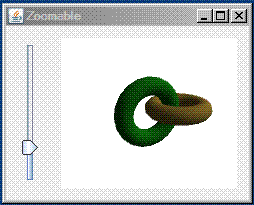## Form application

We put the graphics on a from with a zoom-slider

Main parts of The main class. Note that this is a code extract that only shows the functional parts. It will not compile if copied as is. See versioned project at the end of the module for full source.

_TorusAgain.java

The renderer:

_GLRenderer.java
References
• The animated, mousedriven project: https://svn.hiof.no/svn/psource/JOGL/torus
• The form based project: https://svn.hiof.no/svn/psource/JOGL/torusAgain
Maintainance
Modified may 2010, B Stenseth
(Welcome) JOGL>Torus (Textured Box)